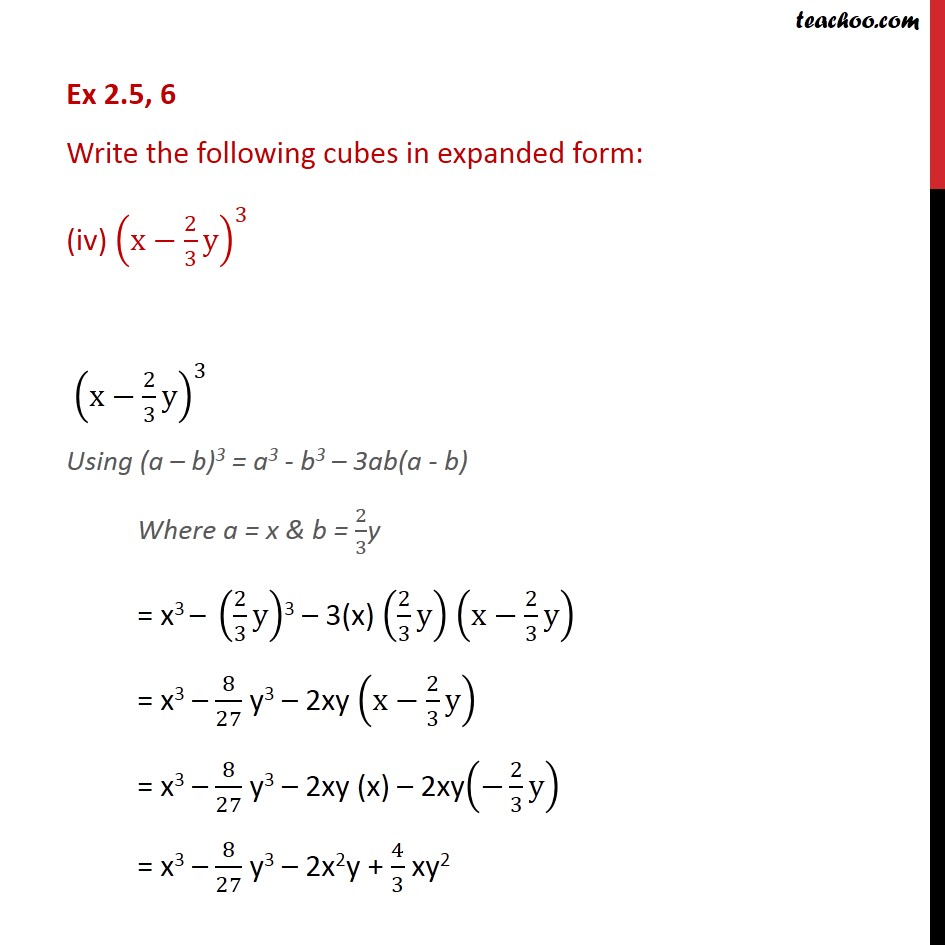1. Chapter 2 Class 9 Polynomials (Term 2)
2. Serial order wise
3. Ex 2.5

Transcript

Ex 2.5, 6 Write the following cubes in expanded form: (iv) (x 2/3 y)^3 (x 2/3 y)^3 Using (a b)3 = a3 - b3 3ab(a - b) Where a = x & b = 2/3y = x3 (2/3 y)3 3(x) (2/3 y) (x 2/3 y) = x3 8/27 y3 2xy (x 2/3 y) = x3 8/27 y3 2xy (x) 2xy( 2/3 y) = x3 8/27 y3 2x2y + 4/3 xy2